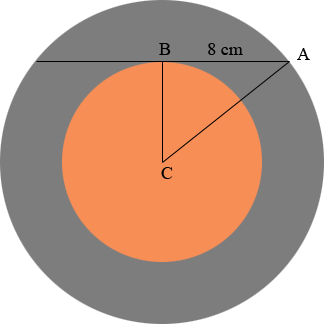SEARCH HOMEMath Central Quandaries & QueriesQuestion from Carol, a student: Good day! Here is a picture of the problem that we need to solve. (I send the picture through e-mail.) A small circle is inside a larger circle, the only given in the problem is the chord of the larger circle tangent to the smaller circle which measures 16cm. The question is, what is the area of the shaded region? Can you answer this question? Thanks! :)Hi Carol,

We didn't get your picture but I think the circles have the same centre and the diagram looks like this.A drew in radii of the two circles and labeled some points. You are to find the area of the region shaded gray, the region between the two circles.

Let R be the radius of the larger circle and r be the radius of the smaller circle. Use the formula for the area of a circle to write an expression for the area of the gray region. Write the expression for Pythagoras theorem applied to the right triangle ABC.

Does this help?
HarleyMath Central is supported by the University of Regina and The Pacific Institute for the Mathematical Sciences.